# Excel XMATCH function with formula examples

The tutorial introduces the new Excel XMATCH function and shows how it is better than MATCH for solving a few common tasks.

In Excel 365, the XMATCH function was added to supersede the MATCH function. But before you start upgrading your existing formulas, it would be wise to understand all advantages of the new function and how it differs from the old one.

In summary, the XMATCH function is same as MATCH but more flexible and robust. It can look up both in vertical and horizontal arrays, search first-to-last or last-to-first, find exact, approximate and partial matches, and use a faster binary search algorithm.

## Excel XMATCH function

The XMATCH function in Excel returns the relative position of a value in an array or a range of cells.

It has the following syntax:

XMATCH(lookup_value, lookup_array, [match_mode], [search_mode])

Where:

Lookup_value (required) - the value to look for.

Lookup_array (required) - the array or range of cells where to search.

Match_mode (optional) - specifies which match type to use:

• 0 or omitted (default) - exact match
• -1 - exact match or the next smallest value
• 1 - exact match or the next largest value
• 2 - wildcard match (*, ?)

Search_mode (optional) - specifies the search direction and algorithm:

• 1 or omitted (default) - search from first to last.
• -1 - search in reverse order from last to first.
• 2 - binary search ascending. Requires lookup_array to be sorted in ascending order.
• -2 - binary search descending. Requires lookup_array to be sorted in descending order.

Binary search is a faster algorithm that works efficiently on sorted arrays. For more info, please see Search mode.

### Which Excel version has XMATCH?

The XMATCH function is only available in Excel for Microsoft 365 and Excel 2021. In Excel 2019, Excel 2016 and earlier versions, this function is not supported.

## Basic XMATCH formula in Excel

To get a general idea of what the function is capable of, let's build an XMATCH formula it its simplest form, defining only the first two required arguments and leaving the optional ones to their defaults.

Supposing, you have a list of oceans ranked by their size (C2:C6) and you wish to find the rank of a particular ocean. To have it done, simply use the ocean's name, say Indian, as the lookup value and the entire list of names as the lookup array:

`=XMATCH("Indian", C2:C6)`

To make the formula more flexible, input the ocean of interest in some cell, say F1:

`=XMATCH(F1, C2:C6)`

As the result, you get an XMATCH formula to look up in a vertical array. The output is the relative position of the lookup value in the array, which in our case corresponds to the ocean's rank: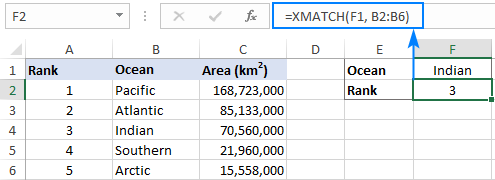A similar formula works perfectly for a horizontal array too. All you need to do is to adjust the lookup_array reference:

`=XMATCH(B5, B1:F1)`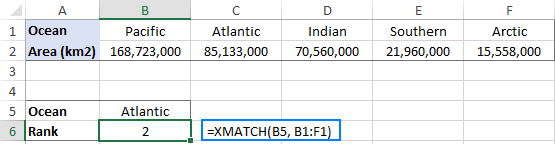### Excel XMATCH function - things to remember

To effectively use XMATCH in your worksheets and prevent unexpected results, please remember these 3 simple facts:

• If there are two or more occurrences of the lookup value in the lookup array, the position of the first match is returned if the search_mode argument is set to 1 or omitted. With search_mode set to -1, the function searches in reverse order and returns the position of the last match as demonstrated in this example.
• If the lookup value is not found, a #N/A error occurs.
• The XMATCH function is case-insensitive by nature and cannot distinguish the letter case. To distinguish lowercase and uppercase characters, use this case-sensitive XMATCH formula.

## How to use XMATCH in Excel - formula examples

The following examples will help you gain more understanding about the XMATCH function and its practical uses.

### Exact match vs. approximate match

The matching behavior of XMATCH is controlled by the optional match_mode argument:

• 0 or omitted (default) - the formula only searches for exact match. If an exact match is not found, a #N/A error is returned.
• -1 - the formula searches for exact match first, and then for the next smaller item.
• 1 - the formula searches for exact match first, and then for the next larger item.

And now, let's see how different match modes affect the formula's result. Suppose you want to find out where a certain area, say 80,000,000 km2, stands among all the oceans.

#### Exact match

If you use 0 for match_mode, you'll get a #N/A error, because the formula cannot find a value exactly equal to the lookup value:

`=XMATCH(80000000, C2:C6, 0)`

#### Next smallest item

If you use -1 for match_mode, the formula will return 3, because the closest match smaller than the lookup value is 70,560,000, and it is the 3rd item in the lookup array:

`=XMATCH(80000000, C2:C6, -1)`

#### Next largest item

If you use 1 for match_mode, the formula will output 2, because the nearest match larger than the lookup value is 85,133,000, which is the 2nd item in the lookup array:

`=XMATCH(80000000, C2:C6, -1)`

The image below shows all the results: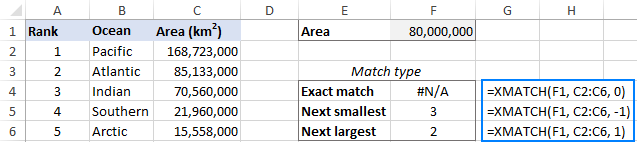### How to match partial text in Excel with wildcards

The XMATCH function has a special match mode for wildcards: the match_mode argument set to 2.

In the wildcard match mode, an XMATCH formula accepts the following wildcard characters:

• Question mark (?) to match any single character.
• Asterisk (*) to match any sequence of characters.

Please keep in mind that wildcards only work with text, not numbers.

For instance, to find the position of the first item that starts with "south", the formula is:

`=XMATCH("south*", B2:B6, 2)`

Or you can type your wildcard expression in some cell, say F1, and supply the cell reference for the lookup_value argument:

`=XMATCH(F1, B2:B6, 2)`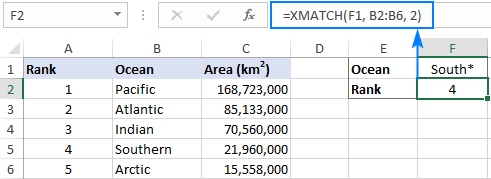With most Excel functions, you'd use tilde (~) to treat the asterisk (~*) or question mark (~?) as literal characters, not wildcards. With XMATCH, tilde is not needed. If you don't define the wildcard match mode, XMATCH will assume that ? and * are regular characters.

For example, the below formula will search the range A2:A7 exactly for the asterisk character:

`=XMATCH("*", A2:A7)`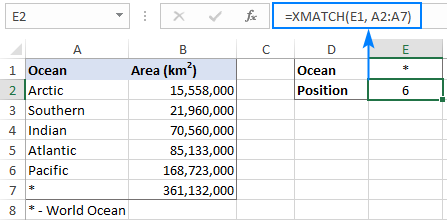In case there are several occurrences of the lookup value in the lookup array, you may sometimes need to get the position of the last occurrence.

The direction of search is controlled be the 4th argument of XMATCH named search_mode. To search in reverse order, i.e. from bottom to top in a vertical array and from right to left in a horizontal array, search_mode should be set to -1.

In this example, we will return the position of the last record for a specific lookup value (please see the screenshot below). For this, set up the arguments as follows:

• Lookup_value - the target salesperson in H1
• Lookup_array - salesperson names in C2:C10
• Match_mode is 0 or omitted (exact match)
• Search_mode is -1 (last-to-first)

Putting the four arguments together, we get this formula:

`=XMATCH(H1, C2:C10, 0, -1)`

Which returns the number of the last sale made by Laura: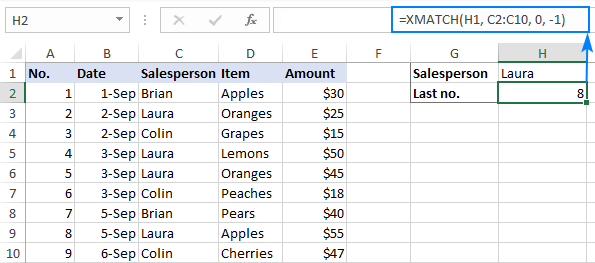### How to compare two columns in Excel for match

To compare two lists for matches, you can use the XMATCH function together with IF and ISNA:

IF( ISNA( XMATCH(target_list, search_list, 0)), "No match", "Match")

For example, to compare List 2 in B2:B10 against List 1 in A2:A10, the formula takes the following form:

`=IF(ISNA(XMATCH(B2:B10, A2:A9)), "", "Match in List 1")`

In this example, we identify only matches, therefore the value_if_true argument of the IF function is an empty string ("").

Enter the above formula in the topmost cell (C2 in our case), press Enter, and it will "spill" into the other cells automatically (it is called a spill range):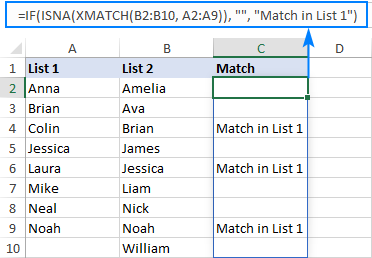How this formula works

At the heart of the formula, the XMATCH function searches for a value from List 2 within List 1. If a value is found, its relative position is returned, otherwise a #N/A error. In our case, the result of XMATCH is the following array:

`{#N/A;#N/A;2;#N/A;4;#N/A;#N/A;8;#N/A}`

This array is "fed" to the ISNA function to be checked for #N/A errors. For each #N/A error, ISNA returns TRUE; for any other value - FALSE. As the result, it produces the following array of logical values, where TRUE's represent non-matches, and FALSE's represent matches:

`{TRUE;TRUE;FALSE;TRUE;FALSE;TRUE;TRUE;FALSE;TRUE}`

The above array goes to the logical test of the IF function. Depending on how you configured the last two arguments, the formula will output the corresponding text. In our case, it's an empty string ("") for non-matches (value_if_true) and "Match in List 1" for matches (value_if_false).

Note. This formula only works in Excel 365 and Excel 2021 that support dynamic arrays. If you are using Excel 2019, Excel 2016 or earlier version, please check out other solutions: How to compare two columns in Excel.

### INDEX XMATCH in Excel

XMATCH can be used in combination with the INDEX function to retrieve a value from another column associated with the lookup value, just like the INDEX MATCH formula. The generic approach is as follows:

INDEX (return_array, XMATCH (lookup_value, lookup_array)

The logic is very straightforward and easy to follow:

The XMATCH function calculates the relative position of the lookup value in the lookup array and passes it to the row_num argument of INDEX. Based on the row number, the INDEX function returns a value from any column that you specify.

For example, to look up the area of the ocean in E1, you can use this formula:

`=INDEX(B2:B6, XMATCH(E1, A2:A6))`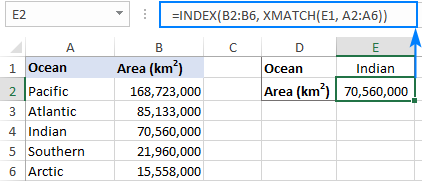### INDEX XMATCH XMATCH to perform 2-dimentional lookup

To look up in columns and rows simultaneously, use INDEX together with two XMATCH functions. The first XMATCH will get the row number and the second one will retrieve the column number:

INDEX (data, XMATCH (lookup_value, vertical_lookup_array), XMATCH (lookup value, horizontal_lookup_array))

The formula is similar to INDEX MATCH MATCH except that you can omit the match_mode argument since it defaults to exact match.

For instance, to retrieve a sales number for a given item (G1) in a specific month (G2), the formula is:

`=INDEX(B2:D8, XMATCH(G1, A2:A8), XMATCH(G2, B1:D1))`

Where B2:D8 are data cells excluding row and column headers, A2:A8 is a list of items and B1:D1 are month names.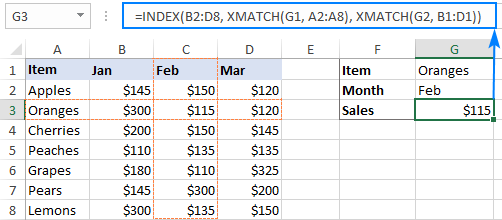### Case-sensitive XMATCH formula

As already mentioned, the Excel XMATCH function is case-insensitive by design. To force it to distinguish text case, use XMATCH in combination with the EXACT function:

MATCH(TRUE, EXACT(lookup_array, lookup_value))

To search in reverse order from last to first:

MATCH(TRUE, EXACT(lookup_array, lookup_value), 0, -1)

The following example shows this generic formula in action. Supposing you have a list of case-sensitive product id's in B2:B11. You are looking to find the relative position of the item in E1. A case-sensitive formula in E2 is as simple as this:

`=XMATCH(TRUE, EXACT(B2:B11, E1))`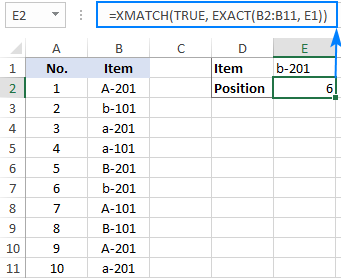How this formula works:

The EXACT function compares the lookup value against each item in the lookup array. If the compared values are exactly equal, including the characters case, the function returns TRUE, FALSE otherwise. This array of logical values (where TRUE's represent exact matches) goes to the lookup_array argument of XMATCH. And because the lookup value is TRUE, the XMATCH function returns the position of the first found exact match or the last exact match, depending on how you configured the search_mode argument.

## XMATCH vs. MATCH in Excel

XMATCH was designed as a more powerful and versatile replacement for MATCH, and so these two functions have a lot in common. However, there are essential differences.

### Different default behavior

The MATCH function defaults to exact match or the next smallest item (match_type set to 1 or omitted).

The XMATCH function defaults to exact match (match_mode set to 0 or omitted).

### Different behavior for approximate match

When the match_mode / match_type argument is set to 1:

• MATCH searches for exact match or next smallest. Requires that the lookup array shall be sorted in ascending order.
• XMATCH searches for exact match or next largest. Does not require any sorting.

When the match_mode / match_type argument is set to -1:

• MATCH searches for exact match or next largest. Requires sorting the lookup array in descending order.
• XMATCH searches for exact match or next smallest. Does not require any sorting.

### Wildcard search

To find partial matches with XMATCH, you need to set the match_mode argument to 2.

The MATCH function does not have a special wildcard match mode option. In most cases, you'll configure it for exact match (match_type set to 0), which also works for wildcard searches.

### Search mode

Like the new XLOOKUP function, XMATCH has a special search_mode argument that allows you to define the direction of search:

• 1 or omitted (default) - search first-to-last.
• -1 - reverse search last-to-first.

And choose a binary search algorithm, which is very quick and efficient on sorted data.

• 2 - binary search on data sorted ascending.
• -2 - binary search on data sorted descending.

Binary search, also called half-interval search or logarithmic search, is a special algorithm that finds the position of a lookup value within an array by comparing it to the middle element of the array. A binary search is much faster than a regular search but works correctly only on sorted lists. On unsorted data, it can return wrong results that may look pretty normal at first sight.

The syntax of MATCH does not provide for the search mode argument at all.

### XMATCH handles arrays natively

Unlike its predecessor, the XMATCH function was designed for dynamic Excel and handles arrays natively, without you having to press Ctrl + Shift + Enter. This makes formulas much easier to build and edit, especially when using a few different functions together. Just compare the following solutions:

### XMATCH and MATCH availability

XMATCH is a new function and it is only available in Excel for Microsoft 365 and Excel 2021.

The MATCH function is available in any version of Excel 365 through Excel 2007.

That's how to use the XMATCH function in Excel. I thank you for reading and hope to see you on our blog next week!

Excel XMATCH formula examples (.xlsx file)

## You may also be interested in

1. MATCH could previously search both vertically and horizontally... minor point, but wanting to share.

• (...for clarification, previously could and still can)

2. I thought perhaps xmatch was my answer but now I'm confused. I'm currently trying to look at values in column G, then based on the value, place another value in another column. I've been working with all of these and they seem to work ok but I can't nest them to continue searching for text and matching it with the value it needs to be. I need values in A2 containing "DC01" to = WADataCenter, values containing "DC02" to = TXDataCenter, values containing "DC03" to = FLDataCenter, values containing "DC04" to = NVDataCenter, and values that contain "DC04" to = "MexicoDataCenter". Kind of like search and replace but I want to keep the data in A2 unchanged.

None of these will nest for me. I tried xmatch as well and could not get that working.
=IFERROR(REPLACE(G2, SEARCH("DC02", A2), 0, "TXDataCenter"), "")'
=IF(OR(ISNUMBER(SEARCH("DC01", A2)), ISNUMBER(SEARCH("WA", A2))), "WADataCenter", "")'
=IF(OR(ISNUMBER(SEARCH("DC01", A2)), ISNUMBER(SEARCH("WA", A2))), "WADataCenter", "")'

3. Dear Svetlana Cheusheva,
i couldn't able to convert a date (MMDDYY) to (MMDDYYY) format in excel. i just google regarding my query then i found some formula as "=DATE(RIGHT(A2,2)&20,MID(A2,3,4),LEFT(A2,2)) " in ablebit.com. Even it's not showing an exact date. Here the example :
A2 Cell Data : 091320 (MMDDYY)
Used formula :=DATE(RIGHT(A2,2)&20,MID(A2,3,4),LEFT(A2,2))
Result: 4/7/2138.
Please advise how do i write formula in order to get valid date as MMDDYYYY.

• Hello Ram,

I cannot find the formula you mentioned on our blog. Most likely you came across the below one that shows how to convert a string or number to a date:
=DATE(RIGHT(A2,4), MID(A2,3,2), LEFT(A2,2))

However, this formula is not suited for your task. It converts a numeric string or number corresponding to a date in the DDMMYYYY format into a serial number representing a date. For example, if you had 13092020 in A2, the formula would convert it into 9/13/2020.

In your case, the first thing to check is what kind of value you have in A2. For this, just look at the Number Format box on the Home tab in the Number group. If it's a date, then simply change the Date format to MMDDYYYY.

If it's a string, then use the following formula:
=DATE("20"&RIGHT(A2,2), LEFT(A2,2), MID(A2,3,2))

Please note, the formula implies that all the dates are in the 21st century - to get the year, we concatenate 20 and the last 2 digits in A2.